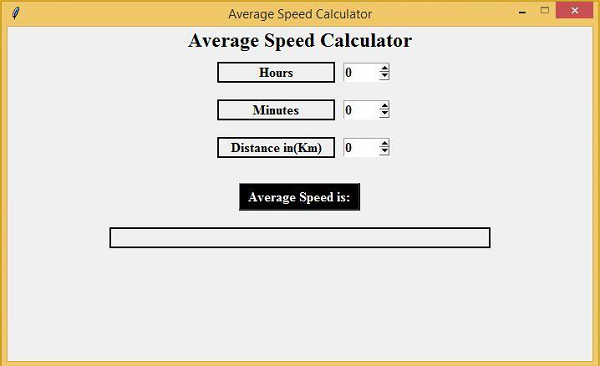# Average Speed Calculator using Tkinter

PythonTkinterGUI-Programming

In this article, we will see how to create a GUI-based application that will calculate the average speed. The average speed of a moving object can be calculated using the following formula,

Average Speed = Distance / [Hours + (Minutes/60)]

To select the input value, we will use the SpinBox method that is used to create a spinner for a range of values. These values are Distance (Kilometers), Hours, and Minutes.

## Example

from tkinter import *
#Create an instance of tkinter frame
win = Tk()

#Set the geometry and resize the frame

win.geometry("700x400")
win.resizable(0,0)
win.title("Average Speed Calculator")
# Create Label for Main Window
Label(win, text="Average Speed Calculator",font=("Times New Roman", 18, "bold"), fg="black").pack()

# Calculate Average Speed
def average_cal():
#hrs
hrs = int(hours.get())
#minutes
mins = int(minutes.get())
#distance
dist = int(distance.get())
#Formula
Used avg = dist/(hrs+(mins/60))
#change the text of label using config method
average_speed.config(text=f"{avg} Km/Hr")
# Create Mulitiple Frames
frame = Frame(win)
frame.pack()

frame1 = Frame(win)
frame1.pack()

frame2 = Frame(win)
frame2.pack()

# Create Labels and Spin Boxes
Label(frame, text="Hours", width=15, font=("Times New Roman", 12, "bold"),borderwidth=2, relief="solid").pack(side=LEFT, padx=10, pady=10) hours = Spinbox(frame, from_=0, to=1000000, width=5,font=("Times New Roman", 12, "bold")) hours.pack(side=LEFT, pady=10)

Label(frame1, text="Minutes", width=15, font=("Times New Roman", 12, "bold"),borderwidth=2, relief="solid").pack(side=LEFT, padx=10, pady=10) minutes = Spinbox(frame1, from_=0, to=10000000, width=5,font=("Times New Roman", 12, "bold")) minutes.pack(side=LEFT, pady=10)

Label(frame2, text="Distance in(Km)", width=15, font=("Times New Roman", 12, "bold"),borderwidth=2, relief="solid").pack(side=LEFT, padx=10, pady=10) distance = Spinbox(frame2, from_=0, to=1000000, width=5,font=("Times New Roman", 12, "bold")) distance.pack(side=LEFT, pady=10)

Button(win, text="Average Speed is:", width=15, font=("Times New Roman", 12, "bold"), command=average_cal, fg="white", bg="black").pack(pady=20)
average_speed = Label(win, text="", width=50, font=("Times New Roman", 12, "bold"), relief="solid") average_speed.pack()

# Execute
Tkinter win.mainloop()

## Output

Running the above code will create and display the Average Calculator.